Next: An example adaptive-step RK4 Up: Integration of ODEs Previous: An example calculation

## Adaptive integration methods

Consider the following system of o.d.e.s: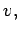(33)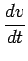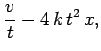(34)

subject to the boundary conditions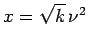and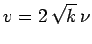at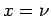, where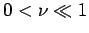. This system can be solved analytically to give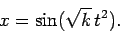(35)

One peculiarity of the above solution is that its variation scale-length decreases rapidly asincreases.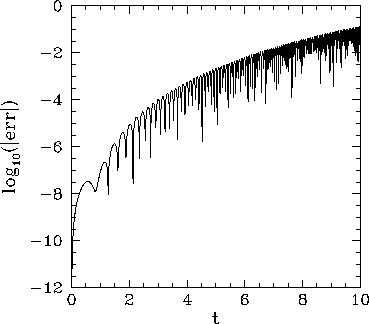Let us compare the above solution with that obtained numerically using a fourth-order Runge-Kutta method. Figure 7 shows the integration error associated with such a method (calculated by integrating the above system, with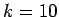, from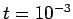to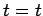, and then taking the difference between the numerical and analytic solutions) with a fixed step-length of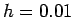, plotted against the independent variable,. It can be seen that, although the error starts off small, it rises rapidly as the variation scale-length of the solution decreases (i.e., asincreases), and quickly becomes unacceptably large. Of course, we could reduce the error by simply reducing the step-length,. However, this is a very inefficient solution. The step-length only needs to be reduced at large. There is no need to reduce it, at all, at small. Clearly, the ideal solution to this problem would be an integration method in which the step-length is varied so as to maintain a relatively constant truncation error per step. Such an adaptive integration method would take large steps when variation scale-length of the solution was large, and vice versa.

Let us investigate how we could convert our fixed step-length, fourth-order Runge-Kutta method into a corresponding adaptive method. First of all, we need an estimate of the truncation error at each step. Suppose that the current step-length is. We can estimate the truncation error,, associated with the current step by taking the difference between the solutions obtained by stepping by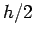twice and byonce (starting from the same point, in both cases). Let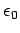be the desired truncation error per step. How do we adjustso as to ensure that the truncation error associated with the next step is closer to this value? Observe, from Eq. (29), that the truncation error per step in a fourth-order scheme scales like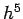. It follows, therefore, that our step-length adjustment formula should take the form16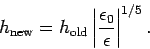(36)

According to this formula, the step-length should be increased if the truncation error per step is too small, and vice versa, in such a manner that the error per step remains relatively constant at.

There are a number of caveats to the above discussion. In a system of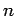coupled o.d.e.s, the overall truncation error per step,, should, of course, be some appropriately weighted average of the errors associated with each equation. There is also a question of whethershould be an absolute error or a relative error. The relative error associated with the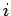th equation is simply the absolute error divided by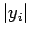, where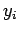is the current value of theth dependent variable. An absolute error estimate is appropriate to a system of equations in which the amplitudes of the various dependent variables remain bounded. A relative error estimate is appropriate to a system in which the amplitudes of the dependent variables blow-up at some point, but the variables always remain the same sign. Finally, a mixed error estimate--usually the minimum of the absolute and relative errors--is appropriate to a system in which the amplitudes of the dependent variables blow-up at some point, but the signs of the variables oscillate. It is usually a good idea to place some limits on the allowed variation of the step-length from step to step: e.g., by preventing the step-length from increasing or decreasing by more than some factor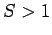per step. This preventsfrom oscillating unduly about its optimum value. Obviously, ifbecomes absurdly small then the integration method has failed, and should abort with an appropriate error message. Finally, a limit should be placed on how largecan become--unfortunately, adaptive methods have a tendency to become a little over optimistic when integration is easy.Figure 8 shows the integration errors associated with a fixed step-length, fourth-order Runge-Kutta method and a corresponding adaptive method--constructed along the lines discussed above--as functions of the independent variable,. The errors are calculated by integrating the current system, with, fromto, and then taking the difference between the numerical and analytic solutions. The fixed step-length associated with the former method is. The desired truncation error per step associated with the latter is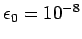. It can be seen that the performance of the adaptive method is far superior to that of the fixed step-length method, since the former method maintains a relatively constant integration error as the variation scale-length of the solution decreases (i.e., asincreases). Figure 9 illustrates how this is achieved. This figure shows the step-length,, associated with the adaptive method as a function of. It can be seen that the adaptive method maintains a relatively constant truncation error per step by decreasingasincreases.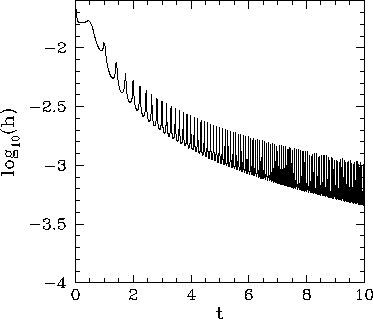Next: An example adaptive-step RK4 Up: Integration of ODEs Previous: An example calculation
Richard Fitzpatrick 2006-03-29